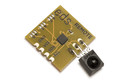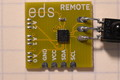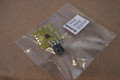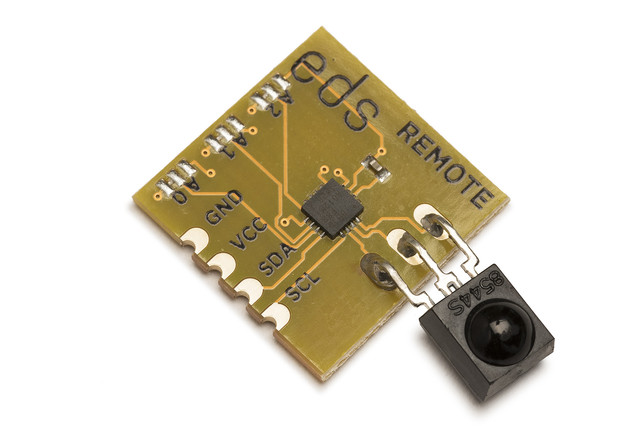# Electric Dollar Store

•••• ## I²C NEC IR Remote Receiver

• 6 meter range
• reports key events with timestamps
• standard 18x18mm module with I²C and power
• solder link selects up to 8 addresses
• Use with ir

• \$1.50

REMOTE combines a sensitive IR receiver with a I²C decoder, to add a remote control function to any project. It continuously scans for IR commands, and reports each one as a 4-byte (32 bit) identifier, with a millisecond timestamp. It also has a simplified function which works with the IR transmitter, reporting any keypresses as ASCII codes. For both receive functions, there is a FIFO of 16 entries. Up to 8 REMOTEs can share an I²C bus under completely independent control.

```import sys
import time

from i2cdriver import I2CDriver, EDS

if __name__ == '__main__':
i2 = I2CDriver(sys.argv, True)

d = EDS.Remote(i2)

print("Press a remote button")
while 1:
k = d.key()
if k is not None:
print("Key     : %r" % k)
r = d.raw()
if r is not None:
(code, timestamp) = r
print("Raw code: %02x %02x %02x %02x (time %.2f)" % (code, code, code, code, timestamp))
```
```#include <Wire.h>

char remote_read()
{
Wire.beginTransmission(0x60);
Wire.write(0);
Wire.endTransmission(false);
Wire.requestFrom(0x60, 1);
return Wire.read();
}

void setup() {
Serial.begin(115200);
Wire.begin();
}

void loop() {
char k = remote_read();
if (k)
Serial.println(k);
}
```
```from machine import I2C
import struct
import time

class Remote:
""" REMOTE is a NEC IR code receiver / decoder """
def __init__(self, i2, a = 0x60):
self.i2 = i2
self.a = a

def regrd(self, addr, fmt = "B"):
b = self.i2.readfrom_mem(self.a, addr, struct.calcsize(fmt))
return struct.unpack(fmt, b)

def key(self):
"""
For the electricdollarstore IR transmitter.
If there is a code in the queue, return its character code.
The layout of the remote is

p     c     n
<     >    ' '
-     +     =
0     %     &
1     2     3
4     5     6
7     8     9

If there is no IR code in the queue, return None.
"""
(r,) = self.regrd(0)
if r != 0:
return chr(r)

def raw(self):
"""
If there is a code in the queue, return a tuple containing the four-byte code,
and a timestamp.
If there is no IR code in the queue, return None.
"""

r = self.regrd(1, "4BH")
if r[:4] != (0xff, 0xff, 0xff, 0xff):
age_in_ms = r
return (r[:4], time.time() - age_in_ms * .001)
else:
return None

def main():
i2 = I2C(1, freq = 100000)

d = Remote(i2)

print("Press a remote button")
while 1:
k = d.key()
if k is not None:
print("Key     : %r" % k)
r = d.raw()
if r is not None:
(code, timestamp) = r
print("Raw code: %02x %02x %02x %02x (time %.2f)" % (code, code, code, code, timestamp))
```

 Default I²C address `0x60` (`0b1100000`) Current consumption (typ.) 5 mA Vcc 2.2 - 3.6 V